Normal view

# Advanced regression models with SAS and R

Material type:TextPublisher: Boca Raton CRC Press 2019Description: xiii, 310 p. Includes illustrations, reference and indexISBN: 9781138049017DDC classification: 519.536 Summary: Advanced Regression Models with SAS and R exposes the reader to the modern world of regression analysis. The material covered by this book consists of regression models that go beyond linear regression, including models for right-skewed, categorical and hierarchical observations. The book presents the theory as well as fully worked-out numerical examples with complete SAS and R codes for each regression. The emphasis is on model accuracy and the interpretation of results. For each regression, the fitted model is presented along with an interpretation of estimated regression coefficients and prediction of response for given values of predictors. Features: Presents the theoretical framework for each regression. Discusses data that are categorical, count, proportions, right-skewed, longitudinal and hierarchical. Uses examples based on real-life consulting projects. Provides complete SAS and R codes for each example. Includes several exercises for every regression. Advanced Regression Models with SAS and R is designed as a text for an upper-division undergraduate or a graduate course in regression analysis. Prior exposure to the two software packages is desired but not required. https://www.routledge.com/Advanced-Regression-Models-with-SAS-and-R/Korosteleva/p/book/9781138049017
Tags from this library: No tags from this library for this title.Average rating: 0.0 (0 votes)
Item type Current location Item location Collection Call number Status Date due Barcode
Books Vikram Sarabhai Library
General Stacks
Slot 1427 (0 Floor, East Wing) Non-fiction 519.536 K6A2 (Browse shelf) Available 201688
##### Browsing Vikram Sarabhai Library shelves, Shelving location: General Stacks, Collection: Non-fiction Close shelf browser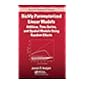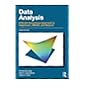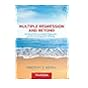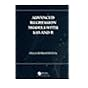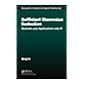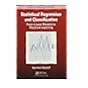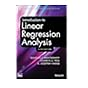519.536 H6R4 Richly parameterized linear models: additive, time series, and spatial models using random effects 519.536 J8D2 Data analysis: a model comparison approach to regression, ANOVA, and beyond 519.536 K3M8 Multiple regression and beyond: an introduction to multiple regression and structural equation modeling 519.536 K6A2 Advanced regression models with SAS and R 519.536 L4S8 Sufficient dimension reduction: methods and applications with R 519.536 M2S8 Statistical regression and classification: from linear models to machine learning 519.536 M6I6 Introduction to linear regression analysis

Table of Contents

Introduction : general and generalized linear regression models
Regression models for response with right-skewed distribution
Regression models for binary response
Regression models for categorical response
Regression models for count response
Regression models for over-dispersed count response
Regression models for proportion response
General linear regression models for repeated measures data
Generalized linear regression model for repeated measures data
Hierarchical regression model.

Advanced Regression Models with SAS and R exposes the reader to the modern world of regression analysis. The material covered by this book consists of regression models that go beyond linear regression, including models for right-skewed, categorical and hierarchical observations. The book presents the theory as well as fully worked-out numerical examples with complete SAS and R codes for each regression. The emphasis is on model accuracy and the interpretation of results. For each regression, the fitted model is presented along with an interpretation of estimated regression coefficients and prediction of response for given values of predictors.
Features:
Presents the theoretical framework for each regression.
Discusses data that are categorical, count, proportions, right-skewed, longitudinal and hierarchical.
Uses examples based on real-life consulting projects.
Provides complete SAS and R codes for each example.
Includes several exercises for every regression.
Advanced Regression Models with SAS and R is designed as a text for an upper-division undergraduate or a graduate course in regression analysis. Prior exposure to the two software packages is desired but not required.

https://www.routledge.com/Advanced-Regression-Models-with-SAS-and-R/Korosteleva/p/book/9781138049017

There are no comments for this item.

to post a comment.
Share

Powered by Koha